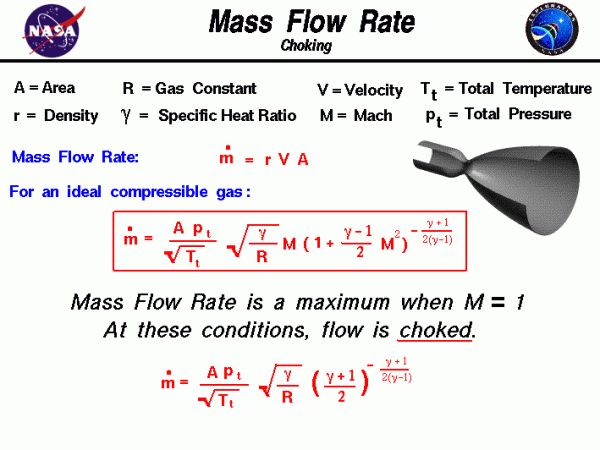# Mass Flow Rate Equations## Mass

The conservation of mass is a fundamental concept of physics. Within some problem domain, the amount of mass remains constant; mass is neither created nor destroyed. The mass of any object is simply the volume that the object occupies times the density of the object. For a fluid (a liquid or a gas) the density, volume, and shape of the object can all change within the domain with time and mass can move through the domain.

The conservation of mass (continuity) tells us that the mass flow rate $$\dot{m}$$ through a tube is a constant and equal to the product of the density r, velocity V, and flow area A:

$$\LARGE \dot{m}=rVA$$

Considering the mass flow rate equation, it appears that for a given area and a fixed density, we could increase the mass flow rate indefinitely by simply increasing the velocity. In real fluids, however, the density does not remain fixed as the velocity increases because of compressibility effects. We have to account for the change in density to determine the mass flow rate at higher velocities. If we start with the mass flow rate equation given above and use the isentropic flow relations and the equation of state, we can derive a compressible form of the mass flow rate equation.

We begin with the definition of the Mach number M, and the speed of sound a:

$$\LARGE V=Ma=M\sqrt{\gamma RT}$$

where gam ($$\bf \gamma$$) is the specific heat ratio, R is the gas constant, and T is the temperature. Now substitute Eq #2 into Eq # 1:

$$\LARGE \dot{m}=rAM\sqrt{\gamma RT}$$

The equation of state is:

$$\LARGE r=\frac{p}{RT}$$

where p is the pressure. Substitute Eq #4 into Eq # 3:

$$\LARGE \dot{m}=AM\sqrt{\gamma RT}\cdot\frac{p}{RT}$$

Collect terms:

$$\LARGE \dot{m}=AM\sqrt{\frac{\gamma}{R}}\cdot\frac{p}{\sqrt{T}}$$

From the isentropic flow equations:

$$\LARGE p=p_{t}(\frac{T}{T_{t}})^{\frac{\gamma}{\gamma-1}}$$

where pt is the total pressure and Tt is the total temperature. Substitute Eq #7 into Eq # 6:

#### Eq # 8

$$\LARGE \dot{m}=\frac{M\frac{Ap_{t}}{\sqrt{T_{t}}}\sqrt{\frac{\gamma}{R}}(\frac{T}{T_{t}})^{\gamma+1}}{2(\gamma-1)}$$

Another isentropic relation gives:

$$\LARGE \frac{T}{T_{t}}=(1+\frac{1}{2}M^{2}(\gamma-1))^{-1}$$

Substitute Eq #9 into Eq # 8:

#### Eq # 10

$$\LARGE \dot{m}=(A\frac{p_{t}}{\sqrt{T_{t}}})\sqrt{\frac{\gamma}{R}}M[1+\frac{1}{2}M^{2}(\gamma-1)]^{-\frac{\gamma+1}{\frac{\gamma-1}{2}}}$$

This equation is shown in the red box on this slide and relates the mass flow rate to the flow area A, total pressure pt and temperature Tt of the flow, the Mach number M, the ratio of specific heats of the gas gam ($$\bf \gamma$$), and the gas constant R: This equation can be further simplified to derive a weight flow function that depends only on the Mach number.

The compressibility effects on mass flow rate have some unexpected results. We can increase the mass flow through a tube by increasing the area, increasing the total pressure, or decreasing the total temperature. But the effect of increasing velocity (Mach number) is a little harder to figure out. If we were to fix the area, total pressure and temperature, and graph the variation of mass flow rate with Mach number, we would find that a limiting maximum value occurs at Mach number equal to one. There is a technique in calculus to find the maximum (or minimum) value of a function by taking the derivative of the function and setting the resulting equation to zero. Let us apply this technique to the mass flow rate equation. To simplify the exercise, let us define:

$$\LARGE B=\sqrt{\frac{\gamma}{R}}\frac{Ap_{t}}{\sqrt{T_{t}}}$$

$$\LARGE C=\frac{\gamma+1}{2(\gamma-1)}$$

$$\LARGE D=\frac{\gamma-1}{2}$$

Then Eq #10 can be written:

$$\LARGE \dot{m}=\frac{BM}{(1+DM^{2})^{C}}$$

We take the derivative of this equation with respect to M and set the result to zero to find the maximum:

#### Eq # 15

$$\LARGE \frac{\text{d}\dot{m}}{\text{d}M}=M\frac{d\frac{1}{(1+DM^{2})^{C}}}{\text{d}M}+\frac{1}{(1+DM^{2})^{C}}=0$$

$$\LARGE \frac{-(2CDM^{2})}{\frac{(1+DM^{2})^{C+1}+1}{(1+DM^{2})^{C}}}=0$$

Using some algebra to simplify this equation:

$$\LARGE 2CDM^{2}=1+DM^{2}$$

Now collect terms which multiply M2:

$$\LARGE M^{2}=\frac{1}{D(2C-1)}$$

We can evaluate the right side of this equation by using Eq #12 and Eq #13:

$$\LARGE D(2C-1)=\frac{1}{2}(\gamma-1)(\frac{\gamma+1}{\gamma-1}-1)=1$$

Therefore, the condition for the maximum airflow occurs at:

$$\LARGE M^{2}=1\text{ or }M=1$$

There is a maximum airflow limit that occurs when the Mach number is equal to one. The limiting of the mass flow rate is called choking of the flow. If we substitute M = 1 into Eq #10 we can determine the value of the choked mass flow rate:

$$\LARGE \dot{M}=(A\frac{p_{t}}{\sqrt{T_{t}}})\sqrt{\frac{\gamma}{R}}(\frac{\gamma+1}{2})^{-\frac{\gamma+1}{\frac{\gamma-1}{2}}}$$

Mach number equal to one is called a sonic condition because the velocity is equal to the speed of sound and we denote the area for the sonic condition by a special symbol “A*”, pronounced “A star”. If we have a tube with changing area, like the nozzle shown on the slide, the maximum mass flow rate through the system occurs when the flow is choked at the smallest area. This location is called the throat of the nozzle. The conservation of mass specifies that the mass flow rate through a nozzle is a constant. If no heat is added, and there are no pressure losses in the nozzle, the total pressure and temperature are also constant.

By substituting the sonic conditions into the mass flow equation in the box, and doing some algebra, we can relate the Mach number M at any location in the nozzle to the ratio between the area A at that location and the area of the throat A*. Since the Mach number is related to the velocity, we can determine the exit velocity of a nozzle, if we know the area ratio from the throat to the exit. Knowing the exit velocity and the mass flow rate, we can determine the thrust of the nozzle. You can explore the operation of a nozzle with our interactive thrust simulator and design your own rockets!

Provide feedback